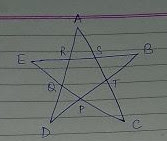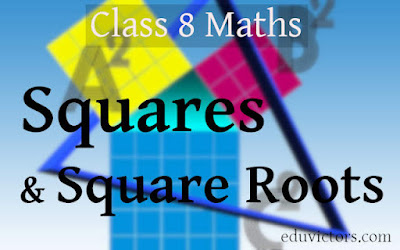## Pages

Showing posts with label Olympiad-maths. Show all posts
Showing posts with label Olympiad-maths. Show all posts

## Tuesday, 13 July 2021

### Class 9 Maths - Important Identities (#class9Maths)(#eduvictors)

Class 9 Maths - Important Identities

(a+b)² = a² + 2ab+ b²

(a–b)² = a² –2ab + b²

a² – b² = (a+b)(a–b)## Lines and Angles - Brain Teaser

Q: What is the measure of ∠A + ∠B + ∠C + ∠D + ∠E  in the figure given below, assuming no angles and sides are equal. Give reason to your answer.## What is so special about Srinivasa Ramanujan's Magic Square?

You may be familiar with magic squares. Srinivasa Ramanujan also designed his own magic square.

This magic square looks similar to any other square. What is so special about it?

Sum of any rows is 139## Friday, 21 September 2012

### CTET Maths (Paper-II)/NTSE SAT/Maths Quiz

CTET Maths (Paper-II)/NTSE SAT/Maths Quiz

Q1: The value of [2 - 3 (2 - 3)-l]-1 is

(a) 5
(b) -5
(c) 1/5
(d) -1/5# Class 8, NTSE - Maths - Square and Square Roots

### Perfect Square

1. A number is called a perfect square if it is expressed as the square of a number.
2. E.g. 1, 4, 9, 16, 25, ... are called perfect squares (1x1 = 1, 2x2 = 4, 3 x 3 = 9...)
3. In square numbers, the digits at the unit’s place are always 0, 1, 4, 5, 6 or 9.
4. The numbers having 2, 3, 7 or 8 at their units' place are not perfect square numbers.

Q1: Which one of the following numbers is a perfect square:
a) 622
b) 393
c) 5778
d) 625

5. If a number ends with the odd number of zeros then it is not a perfect square.

Q2: Check which of the following is not a perfect square.
a) 81000
b) 8100
c) 900
d) 6250000

Answer: a) 81000 (= 92 x 102 x 10)

6. The square of an even number is an even number while the square of an odd number is an odd number.

7. If n is a positive whole number then (n+1)2 - n2 = 2n + 1
or 2n numbers in between the squares of the numbers n and (n + 1)## Monday, 7 November 2011

### NTSE Maths Challenges

Following are the typical problems asked in NTSE, Oympiad Maths,  and other Mathematical based aptitude tests
(best viewed in Chrome or Firefox).

Q1: Ramesh walks from his home to Railway station at the rate of 5 kmph, he misses the train by 7 minutes. However, if he walks at the rate of 6 kmph, he reaches the station 5 minutes before the arrival of the train. Find the distance of the station from his home.

Answer: Let 'd' be the distance between Ramesh's house to Station.
We know that distance = speed x time.

If he walks at speed 5 kmph, let time taken be t1
⇒ t1 = d/5

Let the time t2 is taken when he walks with a speed of 6 kmph.

⇒  t2 = d/6

Since the time gap between two cases is 12 mins = 1/5 hrs
⇒ t1 - t2 = d/5 - d/6 = 1/5 hrs
⇒  6d - 5d = 6
⇒ d = 6km

Q2: Roma travels from place A to B and then from B to C.
From A to B Distance is 55 km which she covers with an average speed of 22 km per hour
It takes her 1 hour 30 minutes moving from B to C with an average speed 30 km per hour
Calculate her average speed over the whole of her journey from A to C.

Answer: We know that $Time = Distance ÷ Speed ∴ Time taken from A to B = 55/22 = 2.5 hours = 2 Hrs and 30 mins Distance from A to B = Speed x Time = 20 x 1.5 = 45 km. (note time taken from B to C is given as 1 Hrs 30 minutes i.e. 1.5 Hrs) Average Speed = Total Distance ÷ Total Time ∴ = (55+45) ÷ (1.5+2.5) = 100 ÷ 4 = 25 kmph## Wednesday, 26 October 2011 ### Maths Challenges (Answers) Maths Challenges (Answers)See Maths Challenges (Questions)... Answer1: When two distinct lines cut each other, they always meet at single point. These two lines can cut the circle maximum at four different points. Therefore, maximum number of intersection points is 5 i.e (d). Following figure explains this. Answer 2: Total possible outcomes are 16 i.e. HHHH, HHHT, HHTH, HHTT, HTHH, HTHT, HTTH, HTTT, THHH, THHT, THTH, THTT, TTHH, TTHT, TTTH, TTTT The catch here is at least as many heads as tails, such possibilities are 11.$\Rightarrow$the probability is$\frac{11}{16}\$

Here product is a prime number. Since we know prime number is divisible by self and number 1 only. So the only choice for m is 1.## Tuesday, 25 October 2011

### Maths Challenges (Questions)

Maths Challenges (Questions)

Q1:A circle and two distinct lines are drawn on a sheet of paper. What is the largest possible number of points of intersection of these figures?
(a) 2        (b) 3      (c)  4    (d) 5

Q2: Reena tosses a coin four times. The probability that she gets at least as many heads as tails is
(a) 5/6      (b) 3/8     (c) 11/16      (d) 5/8

Q3: Given that the product of two whole numbers m × n is a prime number, and the value of m is
smaller than n, find the value of m.

Q4: Jane has 9 boxes with 9 accompanying keys. Each box can only be opened by its accompanying
key. If the 9 keys have been mixed up, find the maximum number of attempts Jane must make
before she can open all the boxes.                     (Singapore Asia-Pacific Mathematical Olympiad Competition)

Q5: If 32 people were to enter a statewide singles tennis tournament, how many matches would be played, including the championship?

Q6: I have 2 vats each containing 2 mats. 2 cats sat on each of the mats. Each cat wore 2 funny old hats. On each hat lay 2 thin rats. On each rat perched 2 black bats. How many things are in my vats? (NCERT class 7 Maths Brain Teasers)

Q7: How many positive integers less than and equal to 999 are divisible by 7 but not by 11?

Q8(SMOPS): How many digits are there before the hundredth 9 in the following number
9797797779777797777797777779......?

Q9(Olympiad): Suppose today is Tuesday. What day of the week shall it be 100 days from now?